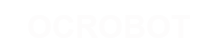#### Site Tools

Action disabled: source

en:learing:examples:ifstatement

# If Statement (Conditional Statement)

The if() statement is the most basic of all programming control structures. It allows you to make something happen or not, depending on whether a given condition is true or not. It looks like this:

```if (someCondition) {
// do stuff if the condition is true
}```

There is a common variation called if-else that looks like this:

```if (someCondition) {
// do stuff if the condition is true
} else {
// do stuff if the condition is false
}```

There's also the else-if, where you can check a second condition if the first is false:

```if (someCondition) {
// do stuff if the condition is true
} else if (anotherCondition) {
// do stuff only if the first condition is false
// and the second condition is true
}```

You'll use if statements all the time. The example below turns on an LED on pin 13 (the built-in LED on many Arduino boards) if the value read on an analog input goes above a certain threshold.

## Hardware Required

 Arduino or Genuino Board Potentiometer or variable resistor Circuit

click the image to enlarge

image developed using Fritzing. For more circuit examples, see the Fritzing project page

Schematic

click the image to enlarge

### Code

In the code below, a variable called analogValue is used to store the data collected from a potentiometer connected to the board on analogPin 0. This data is then compared to a threshold value. If the analog value is found to be above the set threshold the built-in LED connected to digital pin 13 is turned on. If analogValue is found to be < (less than) threshold, the LED remains off.

```/*
Conditionals - If statement

This example demonstrates the use of if() statements.
It reads the state of a potentiometer (an analog input) and turns on an LED
only if the potentiometer goes above a certain threshold level. It prints the analog value
regardless of the level.

The circuit:
* potentiometer connected to analog pin 0.
Center pin of the potentiometer goes to the analog pin.
side pins of the potentiometer go to +5V and ground
* LED connected from digital pin 13 to ground

* Note: On most Arduino boards, there is already an LED on the board
connected to pin 13, so you don't need any extra components for this example.

created 17 Jan 2009
modified 9 Apr 2012
by Tom Igoe

This example code is in the public domain.

http://www.arduino.cc/en/Tutorial/IfStatement

*/

// These constants won't change:
const int analogPin = A0;    // pin that the sensor is attached to
const int ledPin = 13;       // pin that the LED is attached to
const int threshold = 400;   // an arbitrary threshold level that's in the range of the analog input

void setup() {
// initialize the LED pin as an output:
pinMode(ledPin, OUTPUT);
// initialize serial communications:
Serial.begin(9600);
}

void loop() {
// read the value of the potentiometer:
int analogValue = analogRead(analogPin);

// if the analog value is high enough, turn on the LED:
if (analogValue > threshold) {
digitalWrite(ledPin, HIGH);
} else {
digitalWrite(ledPin, LOW);
}

// print the analog value:
Serial.println(analogValue);
delay(1);        // delay in between reads for stability
}```
en/learing/examples/ifstatement.txt · Last modified: 2017/04/07 10:03 (external edit)

### Page Tools